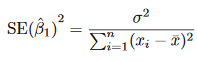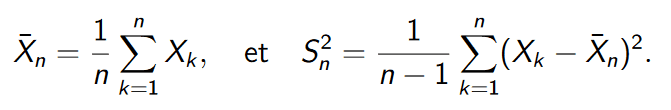# Combining model uncertainties with analytical uncertainties

• A
imsolost
This is my problem : it all starts with some very basic linear least-square to find a single parameter 'b' :
Y = b X + e
where X,Y are observations and 'e' is a mean-zero error term.
I use it, i find 'b', done.

But I need also to calculate uncertainties on 'b'. The so-called "model uncertainty" is needed and I've looked in the reference "https://link.springer.com/content/pdf/10.1007/978-1-4614-7138-7.pdf" where they define the "standard error" : SE(b) as :where sigma² = var(e).
So i have 'b' and var(b). Done.

Now here is the funny part :
I have mutliple sets of observations, coming from monte-carlo samplings on X and Y to account for analytical uncertaines on these observations. So basically, i can do the above stuff for each sample and i have like 1000 samples :
In the end, I have bi and [var(b)]i for i=1,...,1000.

Now the question : what is the mean and the standard deviation ?

What I've done :

1) i have looked in articles that do the same. i found this : "https://people.earth.yale.edu/sites/default/files/yang-li-etal-2018-montecarlo.pdf"
where (§2.3) they face the similar problem, they phrase it very well, but don't explain how they've done it...

2) Imagine you only account for analytical uncertainties. You would get 1000 values of 'b'. It would be easy to calculate a mean and a standard deviation with the common formulas for mean and sample variance. But here i don't have 1000 values ! I have 1000 random variables normally distributed following N(bi, SE(bi)).

3) i looked at multivariate normal distribution. When you have a list of random variables X1, X2,..., here is the expression for the mean and sample variance (basically what I'm looking for, i think) :But i can't find how to calculate this if the X1, X2, ... are not iid (independant and identically distributed), because in my problem, they are independant but they are NOT identically distributed since N(bi, SE(bi)) is different for each 'i'.

4) For the mean it seems to be pretty straightforward and just be the mean of the bi. The standard deviation is the real problem to me.

We need to use the vocabulary of mathematical statistics precisely.

In that vocabulary, a "statistic" is defined by specifying a function of the data in a sample.

3) i looked at multivariate normal distribution. When you have a list of random variables X1, X2,..., here is the expression for the mean and sample variance (basically what I'm looking for, i think) :
View attachment 264285
But i can't find how to calculate this if the X1, X2, ... are not iid (independant and identically distributed),

The expression for ##\overline{X}_n## is the definition of the statistic called the "sample mean" of a sample with values ##X_1,X_2,...X_n##. The expression you give for ##S^2_n## is one possible definition for the "sample variance" of such data. These definitions do not specify any conditions on how the values ##X_1,X_2,...X_n## are generated. In particular they do not say that the each ##X_i## must be an independent realization of a random variable or that each ##X_i## must be an independent realization drawn from distribution of some single random variable ##X##.

Speaking literally, there is no problem calculating those statistics if you have specific set of data. They don't depend on how the data was generated.

A function of random variables is itself a random variable. So if we are in a situation where the values ##x_1,x_2,..,x_n## are regarded as realizations of ##n## random variables, then a statistic ##s(x_1,x_2,...,x_n)## is a random variable. As a random variable ##s## has a distribution. That distribution has parameters. For example, we can speak of "the mean of ##s##" in the sense of the mean of that distribution. If we have ##m## vectors of data of the form ##(x_1, x_2,...x_n)## realized from ##n## random variables, we can compute ##m## values ##s_1, s_2,...s_m## of the random variable ##s##. We can define statistics on such samples. For example, on a sample of ##m## values of ##s##, we defined the statistic called the sample mean.

This shows why discussing even the simplest problems in statistics becomes conceptually complicated. A statistic has its own statistics - in the sense of statistics defined on samples of the statistic. A statistic can be regarded as a random variable and a random variable has a distribution, so a statistic has parameters - in the sense of the parameters of its distribution. Parameters of a distribution are constants, not statistics. But statistics are often used to estimate parameters.

When that is the goal, we call the statistic an "estimator" of the parameter. This is the context where we begin to care about how sets of data ##x_1, x_2,...x_n## are generated - whether they are independent realizations of the same or different random variables, whether the random variables have particular types of distributions - normal distributions or uniform distributions etc.
If we know particular things about the distributions that generate the data, we can say particular things about the distribution of a statistic that is a function of the data.

Now the question : what is the mean and the standard deviation ?

From the above discussion, it should be clear that this question is not precise.

I think you want to know "What statistics are a good estimators for the population mean and population standard deviation (of some random variable) ?".

What random variable are we asking about? That's a subject for another post. Saying the random variable is "##b##" doesn't define it precisely. We need a statement of the probability model for the entire situation.

I have mutliple sets of observations, coming from monte-carlo samplings on X and Y to account for analytical uncertaines on these observations.

Glancing at https://people.earth.yale.edu/sites/default/files/yang-li-etal-2018-montecarlo.pdf , I see the term "analytical" has definition in terms of the physics of a situation. A reader unfamiliar with physics vocabulary could mistake "analytical" for a mathematical term - meaning something based on a particular mathematical formula. What does the term "analytical" indicate about a probability model in your case?

Last edited: